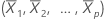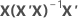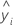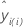# Methods and formulas for diagnostic measures for Stability Study for fixed batches

Select the method or formula of your choice.

## Leverages (Hi)

Leverages are obtained from the hat matrix (H), which is an n x n projection matrix:

The leverage of the ith observation is the ith diagonal element, hi of H. If hi is large, the ith observation has unusual predictors (X1i, X2i, ..., Xpi). That is, the predictor values are far from the mean vector, using Mahalanobis distance.

Leverage values fall between 0 and 1. Minitab identifies observations with leverages over 3p/n or .99, whichever is smaller, with an X in the table of unusual observations. Usually, you examine values with large leverages.

### Notation

TermDescription
Xdesign matrix
hiith diagonal element of the hat matrix
pnumber of terms in the model, including the constant
nnumber of observations

## Cook's distance

Overall measure, D, of the combined impact across all of the estimated regression coefficients on an observation. Minitab calculates D using leverage values and standardized residuals, and considers whether an observation is unusual with respect to both x- and y-values. Observations with large D values may be outliers.

### Formula

Cook's distance is the distance between the coefficients calculated with and without the i th observation. Minitab calculates Cook's distance without fitting a new regression equation each time an observation is omitted. This calculation is:

### Notation

TermDescription
ei i th residual
hi i th diagonal element ofp number of model parameters, including the constant
s 2 mean square error
b coefficient vector
b(i) coefficient vector calculated after deleting the i th observation
X design matrix

## DFITS

Combines leverage and studentized residual (deleted t residuals) values into one overall measure of how unusual an observation is. DFITS measures the influence of each observation on the fitted values in a regression and ANOVA model. Observations with large DFITS values may be outliers.

DFITS represents roughly the number of standard deviations that the fitted value changes when each observation is removed from the data set and the model is refit. Minitab can calculate DFITS without fitting a new regression equation each time an observation is omitted.

### Notation

TermDescription
ei i th residual
hi i th diagonal element ofX design matrixi th fitted responsefitted value calculated without the i th observation
MSE (i) mean square error calculated without the i th observation
n number of observations
p number of model parameters

## Variance inflation factor (VIF)

The VIF can be obtained by regressing each predictor on the remaining predictors and noting the R2value.

### Formula

For predictor xj, the VIF is:

### Notation

TermDescription
R2( xj)coefficient of determination with xj as the response variable and the other terms in the model as the predictors
By using this site you agree to the use of cookies for analytics and personalized content.  Read our policy